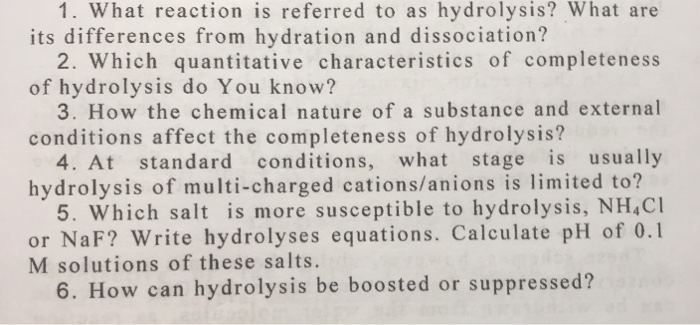# 1. What reaction is referred to as hydrolysis? What are its differences from hydration and dissociation? 2. Which q...1. What reaction is referred to as hydrolysis? What are its differences from hydration and dissociation? 2. Which quantitative characteristics of completeness of hydrolysis do You know? 3. How the chemical nature of a substance and external conditions affect the completeness of hydrolysis? 4. At standard conditions, what stage is usually hydrolysis of multi-charged cations/anions is limited to? 5. Which salt is more susceptible to hydrolysis, NH CI or NaF? Write hydrolyses equations. Calculate pH of 0.1 M solutions of these salts. 6. How can hydrolysis be boosted or suppressed?

1) As a definition we have that hydrolysis is when the water molecule and the reactive molecule are divided into a reaction and its parts are part of another molecule.

A dissociation is when a molecule divides upon contact with water, and a hydration is when the entire water molecule begins to be part of another molecule.

2) In water, the salts dissociate to form ions (completely or incompletely depending on the respective solubility constant, Ks).

The hydrolysis involving ionic compounds can be illustrated by the chemical changes that occur in an aqueous solution of the sodium acetate salt.

3) The temperature directly affects the hydrolysis of a substance, in addition to the equilibrium constant of solubility, acidity, etc., which is respective of each molecule also affects its decomposition.

5) The F- has a greater hydrolysis constant, therefore the substance that is more prone to suffer it, according to the reaction:

F- + H2O = HF + OH-

Second the NH4 +, of the form:

NH4 + + H2O = NH3 + H3O +

The generic expression to calculate the concentration of OH- produced by the hydrolysis of F- and H3O + produced by NH4 +:

[OH- or H3O +] = √ (Kb or Ka) * 0.1 M

Is calculated:

For F-:

[OH-] = √1.39x10 ^ -11 * 0.1 = 1.18x10 ^ -6 M

pOH = - log 1.18x10 ^ -6 = 5.93

pH = 14 - 5.93 = 8.07

For NH4 +:

[H3O +] = √5.6x10 ^ -10 * 0.1 = 7.48x10 ^ -6

pH = - log 7.48x10 ^ -6 = 5.13

6) By manipulating the variables that affect hydrolysis, such as temperature, it can be favored or delayed.

If you liked the answer, please rate it in a positive way, you would help me a lot, thank you.

##### Add Answer of: 1. What reaction is referred to as hydrolysis? What are its differences from hydration and dissociation? 2. Which q...
More Homework Help Questions Additional questions in this topic.